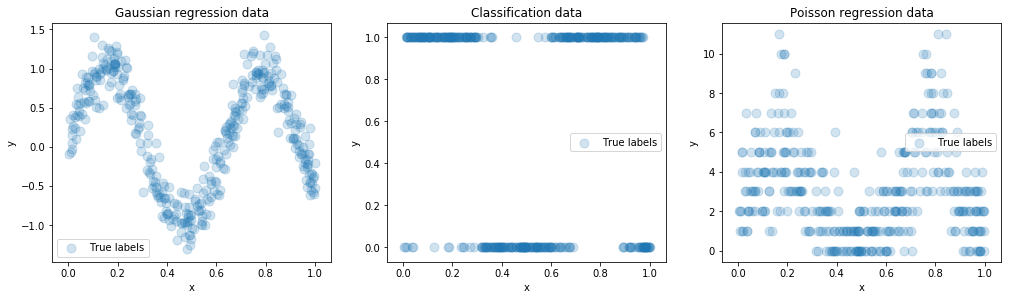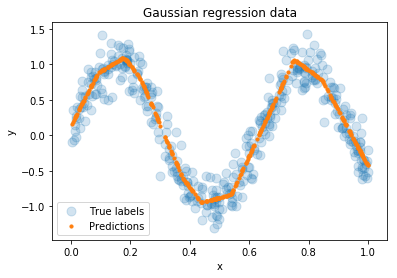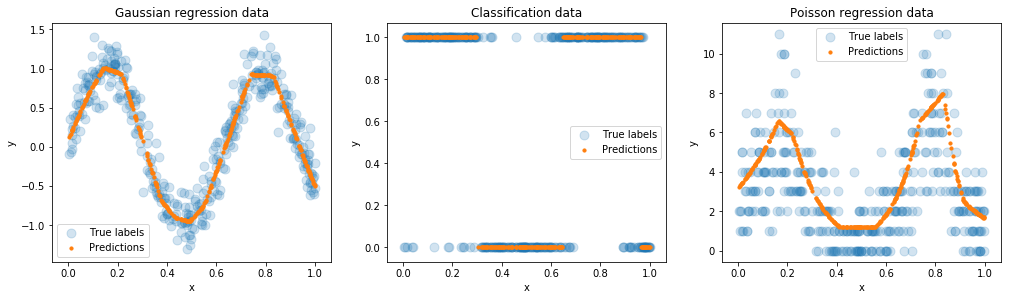# Who needs loss functions? Mixing manual and automatic differentiation

24 May 2018

(This blog post was written as a Jupyter notebook. The .ipynb file of this blog post and associated files can be found here.)

The purpose of this post is to demonstrate that sometimes while using modern deep learning frameworks such as PyTorch or Tensorflow it's useful to not rely wholly on automatic differentiation.

The example application I'll use is regression where the labels/targets, conditional on the input, are sampled from an exponential family distribution, and where we train the network by minimizing the negative log-likelihood of the data. I.e., we'll deal with non-linear Generalized Linear Models (GLMs), or GLMs with learned representations. This encompasses regression with squared loss, Poisson regression, and classification with cross-entropy loss, the three examples I'll use in this post.

I'll show that by doing part of the backpropagation manually, we can avoid explicitly specifying a loss function, and the only thing we'll have to do to switch between label distributions is change the activation function used on the final layer. I'll use PyTorch, but the following can be achieved in TensorFlow.

## Setting up Synthetic Datasets¶

First we need some data. Inputs will be scalar. For regression with squared loss, we'll fit a simple sin wave (with Gaussian noise). For binary classification and Poisson regression we'll fit appropriate transformations of the same data with appropriate error distributions. (Don't worry too much about the code in this block; you can skip right ahead to the plots of the data immediately below.)

In :
import numpy as np
import matplotlib.pyplot as plt
import torch
%matplotlib inline

num_examples = 400

X = np.random.random(num_examples)
X1 = torch.unsqueeze(torch.tensor(X, dtype=torch.float32), 1)
y = np.sin(10 * X)

# Labels for regression with Gaussian noise
gaussian_regression_y = np.random.normal(loc=y, scale=0.2)

# Labels for binary classification (Categorical noise)
class_1_probabilities = 1 / (1 + np.exp(-3.5 * y))
classification_y = np.random.binomial(1, p=class_1_probabilities)
classification_y_one_hot = np.zeros((num_examples, 2))
classification_y_one_hot[np.arange(num_examples), classification_y] = 1

# Labels for Poisson regression
lambdas = 2 * np.exp(y)
poisson_regression_y = np.random.poisson(lam=lambdas)

from collections import OrderedDict

datasets = OrderedDict()
datasets['Gaussian regression'] = {'data': torch.unsqueeze(torch.tensor(gaussian_regression_y, dtype=torch.float32), 1), 'plotting_data': gaussian_regression_y}
datasets['Classification'] = {'data': torch.tensor(classification_y_one_hot, dtype=torch.float32), 'plotting_data': classification_y}
datasets['Poisson regression'] = {'data': torch.unsqueeze(torch.tensor(poisson_regression_y, dtype=torch.float32), 1), 'plotting_data': poisson_regression_y}

def plot_data(regression_type, X, y, predictions=None):
plt.scatter(X, y, s=80, label="True labels", alpha=0.2)
if predictions is not None:
if regression_type == "Classification":
predictions = np.argmax(predictions, axis=1)
plt.scatter(X, predictions, s=10, label="Predictions")
plt.xlabel("x")
plt.ylabel("y")
plt.title("{} data".format(regression_type))
plt.legend()

fig = plt.figure(figsize=(17,4.4))
for data_i, dataset_key in enumerate(datasets.keys()):
data = datasets[dataset_key]['plotting_data']
plot_data(dataset_key, X, data)## Defining the Network¶

Now we'll define a simple, small feed-forward neural network with dense connectivity and ReLU activation functions. We'll use the same neural network for each of our regression problem types.

In :
import torch.nn as nn
import torch.nn.functional as F

class Net(nn.Module):

def __init__(self, output_dim=1):
super(Net, self).__init__()
self.fc1 = nn.Linear(1, 30)
self.fc2 = nn.Linear(30, 20)
self.fc3 = nn.Linear(20, output_dim)

def forward(self, x):
x = F.relu(self.fc1(x))
x = F.relu(self.fc2(x))
x = self.fc3(x)
return x


## A Useful Property of Natural Exponential Family Distributions¶

The Gaussian, Categorical, and Poisson distributions are all instances of the natural exponential family (a subset of the exponential family) of distributions. This means that their probability functions can be expressed as $$q(y) = h(y) \exp{(\eta \cdot y - A(\eta))} \quad ,$$

where $\eta$ is called the natural parameter of the distribution and $A$, the log-partition function, simply normalizes the probability function such that it sums/integrates to $1$. For each function in the exponential family, there exists a canonical link function $f$ which gives the relationship between the natural parameter $\eta$ and the mean of the distribution: $$\mathbb{E}_q[y] = \sum y \cdot q(y) = f^{-1}(\eta) \quad .$$

For example, for labels following a Gaussian distribution, the inverse link function is the identity function. For the Categorical distribution, it's the softmax function (in which case $\eta$ is the vector of logits). For the Poisson distribution, it's the exponential function.

For each of the regression problems dealt with in this post (Gaussian, Categorical, Poisson), the label $y$ is, conditional on the input $x$, sampled from a natural exponential family distribution. I.e., there is some function $\eta(x)$ such that the label $y$ for input $x$ has probability function $$q(y \mid \eta(x)) \quad .$$

Often, what we want to estimate is, conditional on the input $x$, the expected value of the label $\mathbb{E}_q[y]$. Call this estimate $\hat{y}(x)$. This will be the (post-activation) output of our neural network. Suppose we use the inverse link function $f^{-1}$ as the activation function of the final layer of the network. In this case, the pre-activation final layer will be an estimate of the natural parameter, which we'll call $\hat{\eta}(x)$. (I.e., we're talking about fitting Generalised Linear Models, but where the natural parameter estimate $\hat{\eta}$ is a nonlinear function of the inputs.)

Suppose we use the negative log-likelihood of the true labels as a loss function $L$. For a single example with input $x$ and label $y$:

$$L = - \ln q(y \mid \hat{\eta}(x)) = - \ln h(y) - \hat{\eta}(x) \cdot y + A(\hat{\eta}(x)) \quad .$$

In order to do parameter updates by gradient descent, we need the derivatives of the loss with respect to the network parameters, which can be decomposed by the chain rule: $$\frac{\partial L}{\partial \theta} = \frac{\partial L}{\partial \hat{\eta}} \frac{\partial \hat{\eta}}{\partial \theta} \quad,$$

where $\theta$ is a particular network parameter. For every natural exponential family label distribution, the derivative of this loss with respect to the natural parameter is the same:

$$\frac{\partial L}{\partial \hat{\eta}} = \mathbb{E}_\hat{\eta}[y] - y = \hat{y} - y \quad .$$

The upshot of this is that instead of explicitly defining the loss function $L$ to be the negative log-likelihood function for the relevant label distribution and doing backpropagation from the loss, we can instead define $\partial L / \partial \hat{\eta} = \hat{y} - y$ (implicitly defining the loss by our choice of activation function on the final layer) and start backpropagation from the natural parameter estimate layer. Essentially we're doing one step of the backpropagation manually, and relying on auto-differentation for the rest.

## An Example with Gaussian Distributed Labels¶

In the following code, we fit the Gaussian distributed data by explicitly specifying and minimising a mean-squared error loss function (equivalent up to irrelevant constants to the negative log-likelihood for a Gaussian target distribution). We won't worry about evaluating on a validation set.

In :
import torch.optim as optim

In :
torch.manual_seed(500)

net = Net()

y = datasets['Gaussian regression']['data']

optimizer = optim.SGD(net.parameters(), lr=0.2)
loss_function = nn.MSELoss()

for i in range(5000):
eta_hat = net(X1)
y_hat = eta_hat
loss = 0.5 * loss_function(y_hat, y)

loss.backward()
optimizer.step()
if i % 500 == 0:
print("Epoch: {}\tLoss: {}".format(i, loss.item()))

plot_data("Gaussian regression", X, y, y_hat.detach())

Epoch: 0	Loss: 0.23857589066028595
Epoch: 500	Loss: 0.13250748813152313
Epoch: 1000	Loss: 0.07796521484851837
Epoch: 1500	Loss: 0.047447897493839264
Epoch: 2000	Loss: 0.032297104597091675
Epoch: 2500	Loss: 0.02540348283946514
Epoch: 3000	Loss: 0.02224355936050415
Epoch: 3500	Loss: 0.02245643362402916
Epoch: 4000	Loss: 0.022122113034129143
Epoch: 4500	Loss: 0.01919456571340561Compare the above to the result of running the following code, in which instead of doing backpropagation from the loss, we do backpropagation from the natural parameter prediction $\hat{\eta}$ ($\texttt{eta}$ in the code), while setting the accumulated backprop gradient explicitly to $1/\text{batch_size} * (\hat{y} - y)$. Note that we don't need to specify a loss function at all in the following, and we do so only so that the loss can be reported. For optimisation purposes, the loss function has been implicitly set to the negative log-likelihood for the Gaussian distribution by choosing the appropriate inverse link function (the identity function, i.e., the line $\texttt{y_hat} = \texttt{eta_hat}$).

In :
torch.manual_seed(500)

net = Net()

optimizer = optim.SGD(net.parameters(), lr=0.2)
loss_function = nn.MSELoss()

for i in range(5000):
eta_hat = net(X1)
y_hat = eta_hat

# Specifying the loss function is not strictly necessary; it's done here so that the value can be reported
loss = 0.5 * loss_function(y_hat, y)

eta_hat.backward(1.0/num_examples * (y_hat - y))
optimizer.step()
if i % 500 == 0:
print("Epoch: {}\tLoss: {}".format(i, loss.item()))

plot_data("Gaussian regression", X, y, y_hat.detach())

Epoch: 0	Loss: 0.23857589066028595
Epoch: 500	Loss: 0.13250748813152313
Epoch: 1000	Loss: 0.07796521484851837
Epoch: 1500	Loss: 0.047447897493839264
Epoch: 2000	Loss: 0.032297104597091675
Epoch: 2500	Loss: 0.02540348283946514
Epoch: 3000	Loss: 0.02224355936050415
Epoch: 3500	Loss: 0.02245643362402916
Epoch: 4000	Loss: 0.022122113034129143
Epoch: 4500	Loss: 0.01919456571340561We achieve exactly the same results as when explicitly specifying the loss function.

## The General Case¶

The following code demonstrates how easy it is to switch between different types of regression in this way. We pass through the main loop three times, once for regression with Gaussian distributed labels, once for classification, and once for regression with Poisson distributed labels. The only differences between these cases (marked "$\texttt{# ***}$" in the code) are:

• Setting the network output dimension (2 for binary classification, 1 for the regression examples)
• Setting the final layer activation function to be the appropriate inverse canonical link function, which implicitly sets the loss to be minimised to be the negative log-likelihood for the corresponding distribution
In :
datasets['Gaussian regression'].update({'final layer activation': lambda x: x, 'output_dim': 1})
datasets['Classification'].update({'final layer activation': nn.Softmax(dim=1), 'output_dim': 2})
datasets['Poisson regression'].update({'final layer activation': torch.exp, 'output_dim': 1})

fig = plt.figure(figsize=(17,4.4))

for regression_type_i, regression_type in enumerate(datasets.keys()):
y = datasets[regression_type]['data']
plotting_y = datasets[regression_type]['plotting_data']

# *** Difference 2: setting the network output dimension
net = Net(output_dim = datasets[regression_type]['output_dim'])

optimizer = optim.SGD(net.parameters(), lr=0.2)

for i in range(5000):
eta_hat = net(X1)

# *** Difference 3: The inverse of the canonical link function for the
# label distribution is used as the final layer activation function.
y_hat = datasets[regression_type]['final layer activation'](eta_hat)

# Using the appropriate activation above means that the following results in
# implicitly minimizing the negative log-likelihood of the true labels
eta_hat.backward(1.0/num_examples * (y_hat - y))
optimizer.step()

plot_data(regression_type, X, plotting_y, y_hat.detach())## Why?¶

So what are the advantages of this approach?

In the example given here, it means not having to worry about the implementation of the loss function; while modern frameworks such as PyTorch and TensorFlow have efficient and numerically stable implementations of loss functions that are (in terms of optimisation) equivalent to the negative log-likelihood of the most common label distributions, if you want to do regression with a less common label distribution you'll have to write the loss function yourself. For many distributions, there'll be numerical/precision issues along the way.

If your unusual label distribution is a member of the natural exponential family (and there's a good chance that it is), then you can take the approach described above. You'll still need to implement the appropriate inverse link function, but you won't need to worry about its gradient being well behaved.

More generally, it's useful to keep in mind that while auto-differentiation is extremely useful, we don't need to use it all the time and there can be advantages in doing parts of the backpropagation manually.

I've also found the approach described in this post to be a useful trick to simplify the training of an ensemble of neural networks, but I'll cover that in a future post.